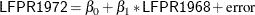Example 94.1 Simple Random Sampling

This example investigates the relationship between the labor force participation rate (LFPR) of women in 1968 and 1972 in large cities in the United States. A simple random sample of 19 cities is drawn from a total of 200 cities. For each selected city, the LFPRs are recorded and saved in a SAS data set Labor. In the following DATA step, LFPR in 1972 is contained in the variable LFPR1972, and the LFPR in 1968 is identified by the variable LFPR1968:

data Labor;
input City \$ 1-16 LFPR1972 LFPR1968;
datalines;
New York        .45     .42
Los Angeles     .50     .50
Chicago         .52     .52
Detroit         .46     .43
San Francisco   .55     .55
Boston          .60     .45
Pittsburgh      .49     .34
St. Louis       .35     .45
Connecticut     .55     .54
Washington D.C. .52     .42
Cincinnati      .53     .51
Baltimore       .57     .49
Newark          .53     .54
Minn/St. Paul   .59     .50
Buffalo         .64     .58
Houston         .50     .49
Patterson       .57     .56
Dallas          .64     .63
;

Assume that the LFPRs in 1968 and 1972 have a linear relationship, as shown in the following model:You can use PROC SURVEYREG to obtain the estimated regression coefficients and estimated standard errors of the regression coefficients. The following statements perform the regression analysis:

title 'Study of Labor Force Participation Rates of Women';
proc surveyreg data=Labor total=200;
model LFPR1972 = LFPR1968;
run;

Here, the TOTAL=200 option specifies the finite population total from which the simple random sample of 19 cities is drawn. You can specify the same information by using the sampling rate option RATE=0.095 (19/200=.095).

Output 94.1.1 summarizes the data information and the fit information.

Output 94.1.1: Summary of Regression Using Simple Random Sampling

 Study of Labor Force Participation Rates of Women

The SURVEYREG Procedure

Regression Analysis for Dependent Variable LFPR1972

Data Summary
Number of Observations 19
Mean of LFPR1972 0.52684
Sum of LFPR1972 10.01000

Fit Statistics
R-square 0.3970
Root MSE 0.05657
Denominator DF 18

Output 94.1.2 presents the significance tests for the model effects and estimated regression coefficients. The F tests and t tests for the effects in the model are also presented in these tables.

Output 94.1.2: Regression Coefficient Estimates

Tests of Model Effects
Effect Num DF F Value Pr > F
Model 1 13.84 0.0016
Intercept 1 4.63 0.0452
LFPR1968 1 13.84 0.0016

 Note: The denominator degrees of freedom for the F tests is 18.

Estimated Regression Coefficients
Parameter Estimate Standard Error t Value Pr > |t|
Intercept 0.20331056 0.09444296 2.15 0.0452
LFPR1968 0.65604048 0.17635810 3.72 0.0016

 Note: The denominator degrees of freedom for the t tests is 18.

From the regression performed by PROC SURVEYREG, you obtain a positive estimated slope for the linear relationship between the LFPR in 1968 and the LFPR in 1972. The regression coefficients are all significant at the 5% level. The effects Intercept and LFPR1968 are significant in the model at the 5% level. In this example, the F test for the overall model without intercept is the same as the effect LFPR1968.